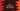# C++ sin() function explanation with example## Example of sin() function in C++ :

In C++, sin() method can be used to find the sine value of a number. It is defined in the C numeric library cmath. cmath contains a lot of other mathematical functions to make it easier for us.

In this tutorial, we will learn how to use sin() function in C++.

### Syntax :

The sin() function is defined as below in C++ 11 :

``````double sin (double x);
float sin (float x);
long double sin (long double x);
double sin (T x);
``````

The last one is for integral type.

### Parameters and Return type :

sin() function takes one argument or the angle in radian. It returns the value of sin() of the argument. The argument and return values are either in double, float or long double.

### Example of using sin() :

``````#include <iostream>
#include <cmath>

#define PI 3.14159265

using namespace std;

int main()
{

cout << "Enter the angle in degree : " << endl; cin >> radian;

cout << "sine of " << radian << " is " << sin(radian * PI / 180) << endl;

return 0;
}
``````

### Sample Output :

``````Enter the angle in degree :
30
sine of 30 is 0.5

Enter the angle in degree :
90
sine of 90 is 1
``````